# Catch up on the latest AI articles# Affine Parameters In Batch Normalization Can Be Detrimental In Few-Shot Learning!

3 main points
✔️ Affine parameters of batch regularization are detrimental in general few-shot transition learning
✔️ Proposed to replace batch regularization by feature regularization with affine parameter removed
✔️ Demonstrate that feature normalization is effective in few-shot transition learning with large domain shift

written by Moslem Yazdanpanah, Aamer Abdul Rahman, Muawiz Chaudhary, Christian Desrosiers, Mohammad Havaei, Eugene Belilovsky, Samira Ebrahimi Kahou
code：The images used in this article are from the paper, the introductory slides, or were created based on them.

## first of all

Batch Normalization, often used in convolutional neural networks, consists of a normalization step for each channel and feature shift scaling with a learnable affine parameter $\gamma,\beta$, which is now an essential part of indispensable in computer vision models.

However, in the paper presented in this article, it is shown that the feature shift scaling process by affine parameters can be detrimental to Few-shot learning. In particular, in the case of Few-shot learning with large domain shift, it was found that removing the affine parameter processing can achieve better performance.

As mentioned earlier, the Batch Normalization (BN) process consists of a per-channel normalization step and feature shift scaling with a learnable affine parameter $\gamma,\beta$.

In our experiments, we study Few-shot learning when the BN layer is replaced by a per-channel normalization step only, in order to investigate the effect of shift scaling due to affine parameters. In the paper, we call this per-channel normalization step Feature Normalization (FN).

#### Definition of Feature Normalization (FN)

To begin, let the domain $\textit(D)$ be the feature space $\textit(X)$ and the surrounding probability distribution $P(X)$(where $X=\{x_1,... ,x_n} \in \textit(X)$).

Let $S$ be a batch consisting of $N$ labeled examples $\{(x^s_i,y^s_i)\}^N_{i=1}$ obtained from the source domain $\textit(D)^s$. Also, for $L$ layers, denote by $\Theta$ the deep convolutional neural network whose $l$-layer weight matrix is $\theta^l$.

Let $h$ be the $l$th intermediate feature of $\Theta$, then the $l$th feature normalization layer is defined for the $c$th channel as follows.

$FN(h_c) = \frac{h_c - \mu_c}{\sqrt{\sigma_{c^2} + \epsilon}}$

where $\mu_c,\sigma_c$ are the first and second order moments of $h_c$, respectively, defined as

$\mu_c = \frac{1}{NHW}\sum_{n,h,w}h_{nchw}$

$\sigma_c = \sqrt{\frac{1}{NHW}\sum_{n,h,w}(h_{nchw} - \mu_c)^2}$

where $H,W$ is the spatial dimension of $h_c$.

#### Fine-tuning affines (Fine-Affine)

In the Few-shot learning setup, we often fit only the linear classifier during fine-tuning and freeze the backbone weights. This is not only to speed up fine-tuning, but also because fine-tuning the backbone does not improve performance (it tends to over-fit).

On the other hand, affine parameters have fewer parameters and may be able to fit the model without overfitting even in the Few-shot learning setup. Therefore, in our experiments, we also introduce a setting where both the linear layer and the affine parameters are jointly fitted (we call this Fine-Affine in the paper). (We call this Fine-Affine in the paper.)

## experimental setup

In our experiments, we investigate the effect of applying FN to state-of-the-art frameworks for few-shot learning such as STARTUP. We also investigate whether replacing BN with FN improves the performance of AdaBN, a BN-based domain adaptation technique, when applied to the framework for few-shot learning.

### benchmark

CDFSL

In our experiments, we use the challenging CDFSL benchmark. As a source dataset, we experiment on miniImageNet or ImageNet consisting of object recognition tasks.

The target dataset consists of four datasets that are composed of completely different domains (with larger domain shifts) than these source datasets.

• EuroSAT (Satellite imagery)
• CropDiseases(Plant Images)
• ChestX (chest X-ray image)
• ISIC2018 (Skin Lesion Imaging)

In our experiments, we perform transition learning in a 5-way k-shot setting where the downstream task has five classes and each class has k samples.

META-DATASET

We also use ImageNet as a base and conduct experiments on META-DATASET.

The target dataset is as follows

• Omniglot
• Aircraft
• Birds
• VGG Flower
• Quickdraw
• Fungi
• Textures
• TrafficSigns
• MSCOCO

A unique feature of this dataset, in addition to the domain shift between source and target, is that the task does not follow the common K-way N-Shot setting.

## experimental results

### Cross-domain few-shot transition learning

First, we compare FN and BN on the CDFSL benchmark. We use ResNet10 for the architecture. The results are as follows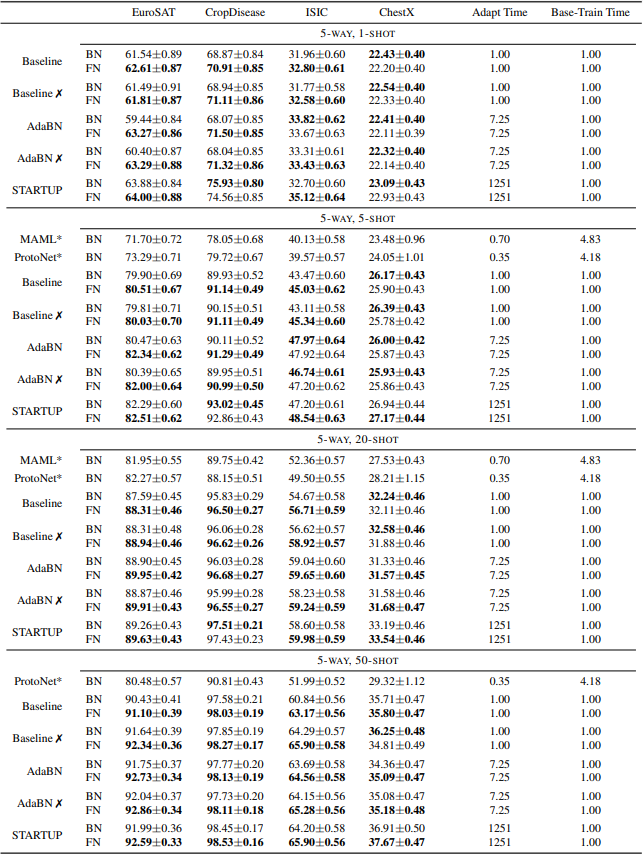Baseline is the standard transition learning setting. The ✗ mark shows the results for the Fine-Affine setting, which jointly fits both the linear layer and the affine parameters. In general, we can see that the average performance of the models with FN outperforms the BN models. The results with META-DATASET are also shown below.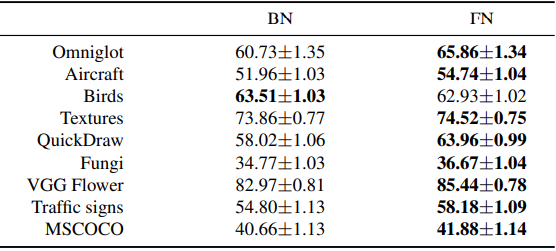Similar to CDFSL, the FN model outperforms the BN model in most cases. These results indicate that for the few-shot transition learning where domain shift occurs, the affine parameter treatment of the BN has a negative impact on the performance.

### Few-shot transition learning between neighboring domains

Next, we investigate the results when the domain shift is small compared to CDFSL. In our experiments, we use miniImageNet as the source and ImageNet as the target. The results are as follows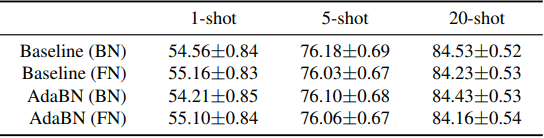The results show that FN does not perform significantly better than BN and that FN is more effective when large domain shifts occur. This can also be seen from the following figure, which evaluates the performance on validation data when trained on ImageNet.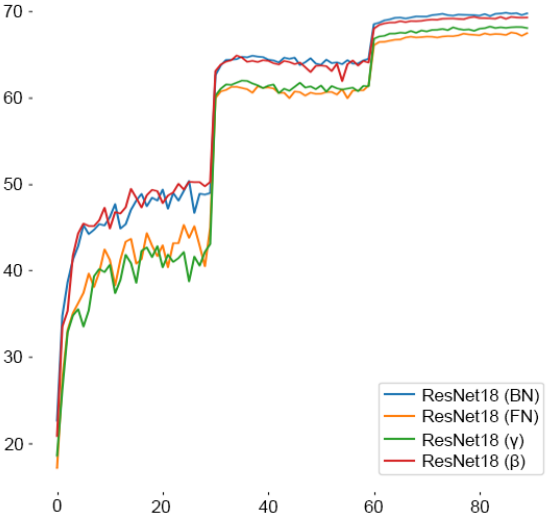In this figure, we can see that BN shows better results than FN in the case of performance evaluation in the domain.

### Fine-Affine setting

The results for fine-tuning both the linear classifier and the affine parameters are also shown below.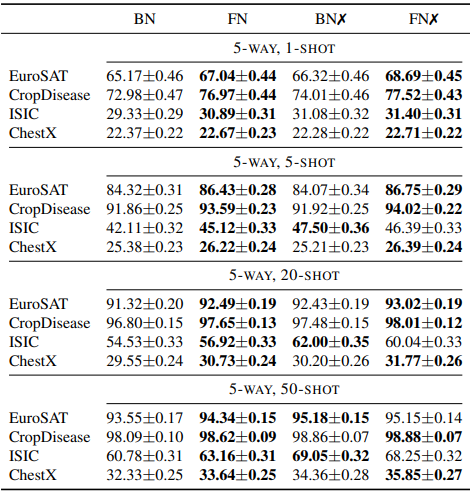The ✗ mark indicates the Fine-Affine setting, where FN is trained in the base learning phase and $\gamma,\beta$ is initialized to 1 and 0, respectively, and introduced during the transition training. In general, we found that both BN and FN perform better in this setting, but the FN model still outperforms the BN model.

### Ablation Research

The BN layer consists of two learnable affine parameters, and the results of removing each of these two ($\gamma,\beta$) parameters are as follows.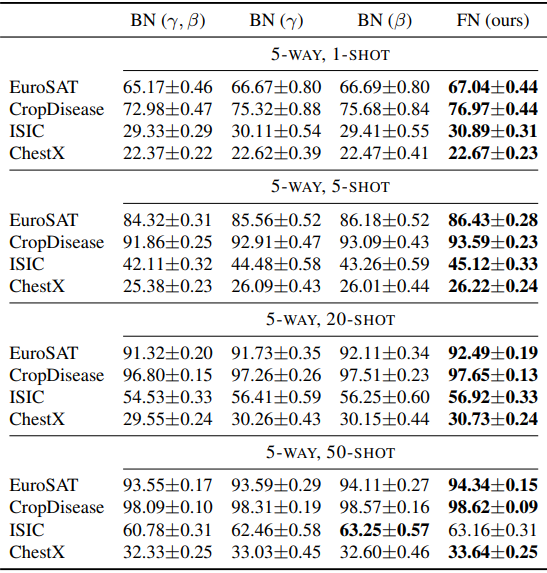In general, $BN(\gamma),BN(\beta)$ with one of the two parameters removed outperforms the regular BN, and FN with both removed shows the best performance.

### Analysis of the differences between FN and BN

Finally, we provide an analysis of the reasons for this difference in results. First, a graphical representation of the distribution of input and output features in the FN and BN layers for large domain shifts is shown below.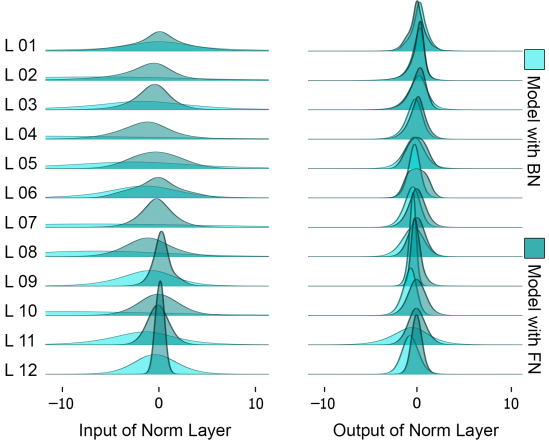As shown in the figure, the input is more centered and the output distribution is relatively similar for FN compared to the BN case, and so on. The paper also assumes that the problem of BN affine parameters under domain shift is related to the sparsification property of ReLU.

To investigate this assumption, we compared sparsity on source and target data.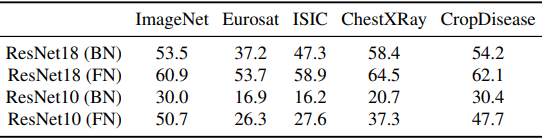In general, the sparsity degradation is suppressed by using FNs for both source and target data. This result suggests that using FNs instead of BNs can suppress the loss of information due to changes in the distribution caused by the threshold properties of ReLUs.

## summary

Although batch normalization is a method applied in various situations, it was found that affine parameters have a negative effect in the case of the few-shot transition learning with a large domain shift. The proposed feature normalization method, which removes the affine parameters, is shown to be effective in solving this problem.

If you have any suggestions for improvement of the content of the article,